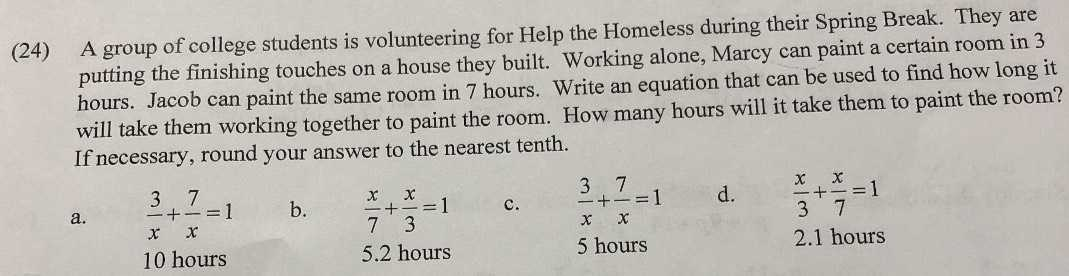### ¿Todavía tienes preguntas de matemáticas?

Pregunte a nuestros tutores expertos
Algebra
Pregunta(24) A group of college students is volunteering for Help the Homeless during their Spring Break. They are putting the finishing touches on a house they built. Working alone, Marcy can paint a certain room in $$3$$ hours. Jacob can paint the same room in $$7$$ hours. Write an equation that can be used to find how long it will take them working together to paint the room. How many hours will it take them to paint the room? If necessary, round your answer to the nearest tenth.

a. $$\frac { 3 } { x } + \frac { 7 } { x } = 1$$ b. $$\frac { x } { 7 } + \frac { x } { 3 } = 1$$ c. $$\frac { 3 } { x } + \frac { 7 } { x } = 1$$ d. $$\frac { x } { 3 } + \frac { x } { 7 } = 1$$

$$10$$ hours $$5.2$$ hours $$5$$ hours $$2.1$$ hours

b. $$\frac { x } { 7 } + \frac { x } { 3 } = 1$$
$$5.2$$ hours